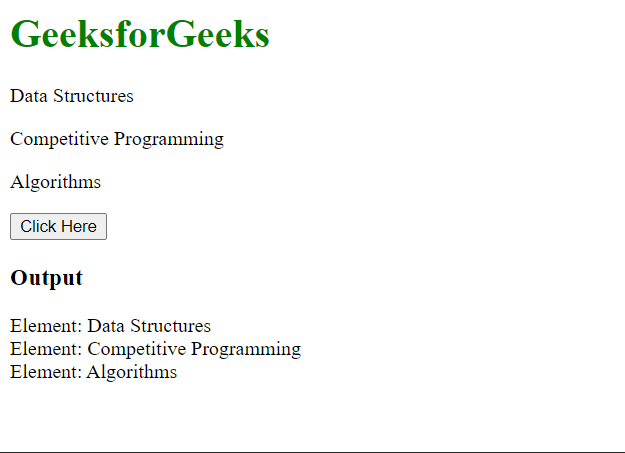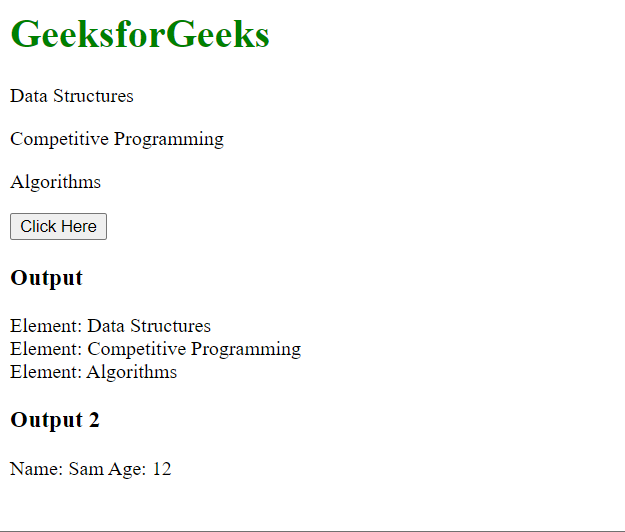# What’s the difference between toArray and makeArray in jQuery ?

• Last Updated : 21 Apr, 2022

In this article, we will learn the difference between the toArray() and makeArray() methods in jQuery.

The toArray() method: This method is used on DOM (Document Object Model) elements to convert them into a JavaScript array. We can iterate this array, calculate its length, and access the elements using their indices like a normal array. However, we cannot create an array from other array-like objects using this method. This is the main difference between the two functions.

Syntax:

`let dom_array = \$("p").toArray();`

Example: In this example, we will see how toArray() can be used to convert DOM elements to an array and how it does not work with other types of data.

## HTML

 `<``html``>``<``head``>``  ``<``script` `src``=``"https://code.jquery.com/jquery-3.6.0.js"``>``  ``````<``body``>``  ``<``h1` `style``=``"color: green;"``>``    ``GeeksforGeeks``  ````  ` `<``p``>Data Structures` `  ` `<``p``>Competitive Programming` `  ` `<``p``>Algorithms`  `  ``<``button``>Click Here``  ``<``h3``>Output``  ` `  ``<``div` `id``=``"output"``>``  ``<``script``>``    ``let obj = {``      ``name: "Sam",``      ``age: 12``    ``}` `    ``\$("button").click(function () {` `      ``// Creating an array of paragraphs``      ``let para_array = \$("p").toArray();` `      ``// Iterating over para_array``      ``// and appending the data in the div``      ``for (i = 0; i < ``para_array.length``; i++) {``        ``\$("#output").append("Element: " +``          ``para_array[i].innerHTML + "
");``      ``}` `      ``// We cannot use toArray() on the object``      ``// as toArray() works only for DOM elements``      ``// let obj_array = jQuery.toArray(obj);``      ``// console.log(obj_array);``    ``});``  ```````

Output:The makeArray() method: This method is used on array-like objects to converts them to an array. We can then use normal array functions on that array. This method supports the conversion of DOM elements as they are array-like objects.

Syntax:

```// Array using DOM elements
let array = jQuery.makeArray(\$("p"));

// Array using other array-like objects
let array2 = jQuery.makeArray(array1, array2, array3);```

Example: In this example, we will see how makeArray() can be used to convert all types of array-like elements including DOM elements.

## HTML

 `<``html``>``<``head``>``  ``<``script` `src``=``"https://code.jquery.com/jquery-3.6.0.js"``>``  ``````<``body``>``  ``<``h1` `style``=``"color: green;"``>``    ``GeeksforGeeks``  ````  `  `<``p``>Data Structures`  `  `  `<``p``>Competitive Programming`  `  `  `<``p``>Algorithms`  `  ``<``button``>Click Here``  ``<``h3``>Output``  ` `  ``<``div` `id``=``"output"``>``  ``<``h3``>Output 2``  ``<``div` `id``=``"output2"``>``  ``<``script``>``    ``let obj = {``      ``name: "Sam",``      ``age: 12``    ``}` `    ``\$("button").click(function () {` `      ``// Creating an array of paragraphs``      ``let para_array = jQuery.makeArray(\$("p"));` `      ``// Iterating over para_array``      ``// and appending the data in the first div``      ``for (let i = 0; i < ``para_array.length``; i++) {``        ``\$("#output").append("Element: " +``          ``para_array[i].innerHTML + "
");``      ``}` `      ``// Creating an array of the object``      ``let obj_array = jQuery.makeArray(obj);` `      ``// Iterating over obj_array``      ``// and appending the data in the second div``      ``for (let i = 0; i < ``obj_array.length``; i++) {``        ``\$("#output2").append("Name: " +``          ``obj_array[i].name + " Age: " +``          ``obj_array[i].age + "
");``      ``}``    ``});``  ```````

Output:Difference between toArray() and makeArray():

My Personal Notes arrow_drop_up